# Intermediate Algebra Tutorial 40

Intermediate Algebra
Tutorial 40: Adding, Subtracting and Multiplying

WTAMU > Virtual Math Lab > Intermediate AlgebraLearning Objectives

After completing this tutorial, you should be able to:Introduction

In this tutorial we will look at adding, subtracting and multiplying radical expressions. If you need a review on what radicals are, feel free to go to Tutorial 37: Radicals.  If it is simplifying radical expressions that you need a refresher on, go to Tutorial 39: Simplifying Radical Expressions.  Ok, I think you are ready to begin this tutorial.Tutorial

The following are two examples of two different pairs of like radicals: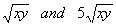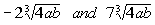If you need a review on simplifying radicals go to Tutorial 39: Simplifying Radical Expressions.

You add or subtract them in the same fashion that you do like terms shown in Tutorial 25: Polynomials and Polynomial Functions. Combine the numbers that are in front of the like radicals and write that number in front of the like radical part.Example 1:   Add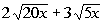.

The 20 in the first radical has a factor that we can take the square root of.

Can you think of what that factor is?

Let's see what we get when we simplify the first radical: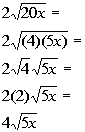*Rewrite 20x as (4)(5x)
*Square root of 4 is 2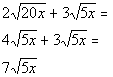Example 2:   Add or subtract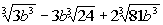.

We can take the cube root of  b cubed in the first radical: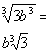*Cube root of b cubed is b

The 24 in the second radical has a factor that we can take the cube root of.

Can you think of what that factor is?

Let's see what we get when we simplify the second radical: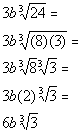*Rewrite 24 as (8)(3)

*Cube root of 8 is 2

We can take the cube root of the b cubed in the third radical and 81 has a factor that we can take the cube root of.

Can you think of what that factor is?

Let's see what we get when we simplify the third radical: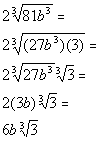*Rewrite 81 as (27)(3)

*Cube root of 27 b cubed is 3b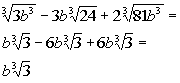Example 3:   Add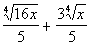.

We can take the fourth root of the 16 in the first radical: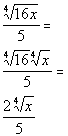*Fourth root of 16 is 2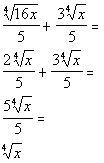Step 1: Multiply the radical expression.

Follow the multiplication property of radicals found in Tutorial 39: Simplifying Radical Expressions and the same basic properties used to multiply polynomials together found in Tutorial 26: Multiplying Polynomials  to multiply radical expressions together.Example 4:   Multiply and simplify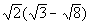.

Step 1: Multiply the radical expression

AND

Using the distributive property found in Tutorial 5: Properties of Real Numbers we get: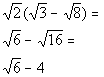*Square root of 16 is 4Example 5:   Multiply and simplify.  Assume variable is positive.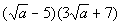Step 1: Multiply the radical expression

AND

We can apply the FOIL method found in Tutorial 26: Multiplying Polynomials to this example: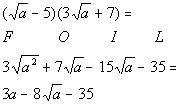*Square root of a squared is aExample 6:   Multiply and simplify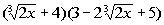.

Step 1: Multiply the radical expression

AND

Using distributive property twice we get: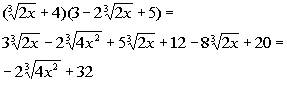Practice Problems

These are practice problems to help bring you to the next level.  It will allow you to check and see if you have an understanding of these types of problems. Math works just like anything else, if you want to get good at it, then you need to practice it.  Even the best athletes and musicians had help along the way and lots of practice, practice, practice, to get good at their sport or instrument.  In fact there is no such thing as too much practice.

To get the most out of these, you should work the problem out on your own and then check your answer by clicking on the link for the answer/discussion for that  problem.  At the link you will find the answer as well as any steps that went into finding that answer.Practice Problems 1a - 1b: Add or subtract.

1a.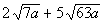1b.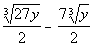Practice Problems 2a - 2b: Multiply and simplify.

2a.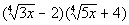2b.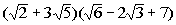Need Extra Help on these Topics?

Go to Get Help Outside the Classroom found in Tutorial 1: How to Succeed in a Math Class for some more suggestions.Last revised on July 21, 2011 by Kim Seward.